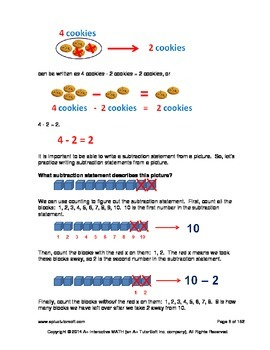# 1st Grade Subtraction Lessons, Worksheets, Solution ManualsSubject
Resource Type
Format
PDF (3 MB|152 pages)
\$3.25
List Price:
\$4.99
You Save:
\$1.74

#### Product Description

Builds a Strong Foundation in Math! This book includes lessons, worksheets and worksheets solution guides. The lessons are easy to understand and teach math concepts with colorful visuals and text. Worksheet for each lesson provides practice in problem solving to master the math concepts. The solution guide for each worksheet provides solution steps with appropriate graphics to further clarify and reinforce math concepts.

The lessons covered in this book are:
* Introduction to Subtraction
* Subtraction Using Objects
* Identity Property of Subtraction
* Subtraction in Relation to Addition
* Subtraction Using a Subtraction Table
* Subtraction Using Regrouping
* Find 10 Less
* True or False
* Find the missing number
* Subtraction without Borrowing Numbers (Optional)
* Subtraction with Borrowing Numbers
* Subtracting a 1-digit Number from a 2-digit Number
* Subtracting a 2-digit Number from a 2-digit Number

Increases student’s confidence level and chances of success in higher level MATH!
Total Pages
152 pages
Included
Teaching Duration
N/A
Report this Resource to TpT
Reported resources will be reviewed by our team. Report this resource to let us know if this resource violates TpT’s content guidelines.
• Ratings & Reviews
• Q & A

Teachers Pay Teachers is an online marketplace where teachers buy and sell original educational materials.